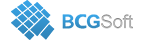BCGSuite for MFC

This enum describes transition types.

Enumerator
TT_UNDEFINED

Undefined.

TT_SUM

formula will calculate sum of similar (located at the same index) data points for all input series.

TT_DIFF

difference

TT_HIGH

high value (max)

TT_LOW

low value (min)

TT_MULTIPLY

multiply

TT_DIVIDE

divide

TT_AVERAGE

average

TT_DIFF_ABS

difference (absolute value)